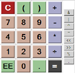# Our Scientific Calculator

In the display window of the calculator below you write the complete mathematical expressions before calculation.

This feature is very handy, especially when working with long complicated formulas.

The values and expressions can be typed by using either keyboard or calculator buttons and the expressions can

be reused and modified by accessing the history tab.

Note! - use radians for trigonometric functions.

##### Constants
• ans : the last calculated result
• PI : pi = 3.14159265...
• E : e = 2.71828182...
• LOG2E : log of e base 2
• LOG10E : log of e base 10
• LN2 : log of 2 base e
• LN10 : log of 10 base e
• SQRT2 : square root of 2
• SQRT1_2 : square root of 1/2
##### Functions
• abs(a) : the absolute value of a
• acos(a) : arc cosine of a
• asin(a) : arc sine of a
• atan(a) : arc tangent of a
• atan2(a,b) : arc tangent of a/b
• ceil(a) : integer closest to a and not less than a
• cos(a) : cosine of a
• exp(a) : exponent of a
• floor(a) : integer closest to and not greater than a
• log(a) : log of a base e
• max(a,b) : the maximu
##### Keyboard shortcuts (Alt or Cmd):
• Clear
• Equals
• History (previous)
• Display (cycle)
• Decimalmixed display
• DecimalScientific display
• Decimal Engineering display
• Binary display
• Octal display
• Hexdisplay

#### Examples

Some simple expressions:

• 23 = pow(2,3) = 8
• log(3) = 1.099
• 2 * (3 + 2) = 10

Note that the expressions can be written in any editor and transferred to the calculator display by using "copy and paste".#### Display

This calculator can handle input numbers in several different bases:

• Decimal (Base 10): numbers that do not start with a zero like 15 or 3.14e15. Decimal numbers can contain digits 0-9, decimals, and scientific notation.
• Hexadecimal (Base 16): Integers that start with a zero x like 0x1a5. Hexadecimal numbers can contain digits 0-9 and a-f (or A-F) but no decimal or scientific notation.
• Octal (Base 8): Integers that start with a zero like 073. Octal numbers can contain digits 0-7 but no decimal or scientific notation.
• Binary (Base 2): Integers that start with a zero b like 0b101. Binary numbers can contain digits 0 and 1 but no decimal or scientific notation.

^ is a bitwise xor operation. To raise a number to a power use pow() function.

Thanks to ostermiller.orgfor providing this calculator under the terms of the GNU General Public License.

### Affiliate Partners

Help support us by using our affiliate links below.